Next: Butterworth band-pass filter Up: Designing filters Previous: Butterworth filters   Contents   Index

Stretching the unit circle with rational functions

In Section 8.3.4 we saw a simple way to turn a low-pass filter into a band-pass one. It is tempting to apply the same method to turn our Butterworth low-pass filter into a higher-quality band-pass filter; but if we wish to preserve the high quality of the Butterworth filter we must be more careful than before in the design of the transformation used. In this section we will prepare the way to making the Butterworth band-pass filter by introducing a class of rational transformations of the complex plane which preserve the unit circle.

This discussion is adapted from [PB87], pp. 201-206 (I'm grateful to Julius Smith for this pointer). There the transformation is carried out in continuous time, but here we have adapted the method to operate in discrete time, in order to make the discussion self-contained.

The idea is to start with any filter with a transfer function as before: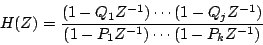whose frequency response (the gain at a frequency) is given by:Now suppose we can find a rational function,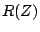, which distorts the unit circle in some desirable way. Forto be a rational function means that it can be written as a quotient of two polynomials (for example, the transfer functionis a rational function). Thatsends points on the unit circle to other points on the unit circle is just the condition thatwhenever. It can easily be checked that any function of the form(where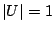) has this property. The same reasoning as in Section 8.2.2 confirms thatwhenever.

Once we have a suitable rational function, we can simply compose it with the original transfer functionto fabricate a new rational function,The gain of the new filterat the frequencyis then equal to that ofat a different frequency, chosen so that:The functionmoves points around on the unit circle;at any point equalson the pointmoves it to.and apply the functionGeometrically, this choice ofstretches the unit circle uniformly to twice its circumference and wraps it around itself twice. The points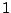andare both sent to the point, and the points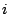andare sent to the point. The resulting transfer function isThe pole-zero plots ofandare shown in Figure 8.19. From a low-pass filter we ended up with a band-pass filter. The pointsandwhichsends to(where the original filter's gain is highest) become points of highest gain for the new filter.Next: Butterworth band-pass filter Up: Designing filters Previous: Butterworth filters   Contents   Index
Miller Puckette 2006-12-30